# Java String join() Method: Complete Guide

Java string join() method returns a string joined with the given delimiter. In the string join method, the delimiter is copied for each element. In the case of a null element, “null” is added.

## Java String join()

Java String join() is a built-in method that is used to join character sequences with a specific delimiter. The delimiter is added between each different character sequence to combine all the character sequences into a single String. To convert an array to string, use the Java String join() method.

See the following figure.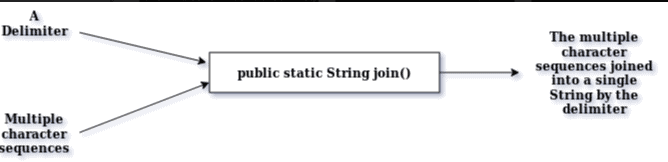See the following syntax.

```public static String join(CharSequence delimiter, CharSequence... elements)

or

public static String join(CharSequence delimiter, Iterable<? Extends CharSequence> elements)
```

### Parameters:

delimiter: This is what the character sequences are joined with each other.

elements: The character sequences in question. This can also be entered utilizing the Collections framework extending Iterable.

### Returns:

The concatenated String.

### Throws:

NullPointerException if the delimiter is null. However, if the character sequence is null, the String “null” is added in place of it.

See the following code example.

```public class Example1 {
public static void main(String[] args) {
String s1 = String.join("+", "One", "One");

System.out.println(s1);
}
}```

#### Output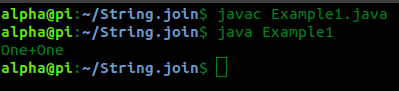Let’s see an example two.

```public class Example2 {
public static void main(String[] args) {
String s1 = String.join(null, "One", "One");

System.out.println(s1);
}
}```

#### Output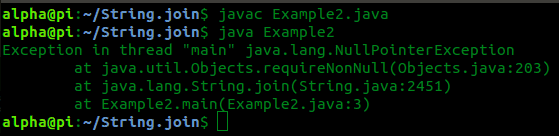In the above example, we got the output NullPointerException.

Let’s see the third example.

```public class Example3 {
public static void main(String[] args) {
String s1 = String.join("+", null, "One");

System.out.println(s1);
}
}```

#### Output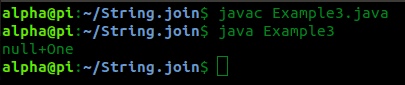See the next example.

```public class Example4 {
public static void main(String[] args) {
String s1 = null;

String s2 = String.join("+", s1, "One");
System.out.println(s2);
}
}
```

#### Output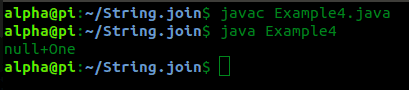The following example demonstrates syntax of the form public static String join(CharSequence delimiter, Iterable<? Extends CharSequence> elements) with the use of an ArrayList.

See the following code.

```import java.util.*;

public class Example5 {
public static void main(String[] args) {

ArrayList<String> arrlst = new ArrayList<>();

String s1 = String.join("+", arrlst);
System.out.println(s1);

}
}
```

#### Output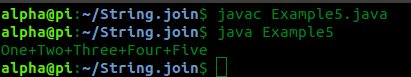That’s it for this tutorial.

## Related Posts

Java string intern()

Java string replace()

Java string replaceAll()

Java string format()

Java string toLowerCase()

This site uses Akismet to reduce spam. Learn how your comment data is processed.